# Gaussian Processes¶

skorch supports integration with the fantastic GPyTorch library. GPyTorch implements various Gaussian Process (GP) techniques on top of PyTorch.

GPyTorch adopts many patterns from PyTorch, thus making it easy to pick up for seasoned PyTorch users. Similarly, the skorch GPyTorch integration should look familiar to seasoned skorch users. However, GPs are a different beast than the more common, non-probabilistic machine learning techniques. It is important to understand the basic concepts before using them in practice.

This notebook is not the place to learn about GPs in general, instead a basic understanding is assumed. If you're looking for an introduction to probabilistic programming and GPs, here are some pointers:

Below, we will show you how to use skorch for Gaussian Processes through GPyTorch. We assume that you are familiar with how skorch and PyTorch work and we will focus on how using GPs differs from using non-probabilistic deep learning techniques with skorch. For a discussion on when and when not to use GPyTorch with skorch, please have a look at our documentation.

If you haven't already, you should install GPyTorch, since it is not installed automatically after installing skorch:

# using pip
pip install -U gpytorch
# using conda
conda install gpytorch -c gpytorch

In :
! [ ! -z "\$COLAB_GPU" ] && pip install torch "skorch>=0.11" gpytorch


## Imports¶

In :
import math
import os
import urllib.request

In :
import numpy as np
import torch
import gpytorch
from matplotlib import pyplot as plt

In :
torch.manual_seed(0)
torch.cuda.manual_seed(0)
plt.style.use('seaborn')
DEVICE = 'cuda' if torch.cuda.is_available() else 'cpu'


# Exact Gaussian Process Regression¶

GPyTorch implmenets different methods to solve GPs. The most basic form is to use exact solutions. Variational GPs are described further below.

## Simple example: sine curve¶

The "Hello world" of GPs is predicting a sine curve with Gaussian noise added on top. We will start with this example.

### Creating the data¶

First we synthesize our data. For training, we use a sine curve with Gaussian noise added on top. For validation, we just use the sine without noise, assuming this is the underlying ground truth. To make it difficult for the model, the training data will only contain very few data points for now.

In :
sampling_frequency = 0.5
X_train = torch.arange(-8, 9, 1 / sampling_frequency).float()
y_train = torch.sin(X_train) + torch.randn(len(X_train)) * 0.2

In :
X_valid = torch.linspace(-10, 10, 100)
y_valid = torch.sin(X_valid)


As you can see below, there is a slight hint of periodicity in the training data but it could also just be noise.

In :
plt.plot(X_train, y_train, 'o')

Out:
[<matplotlib.lines.Line2D at 0x7f522f46d8d0>]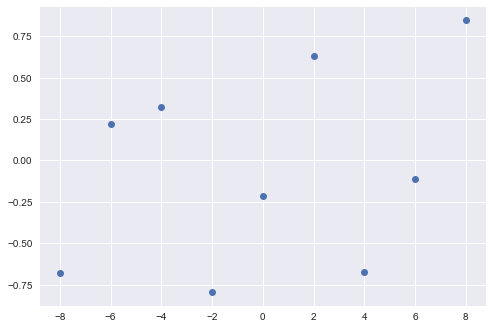### Defining the module¶

As usual with PyTorch, the core of your modeling approach is to define the module. In our case, instead of subclassing torch.nn.Module, we subclass gpytorch.models.ExactGP (which itself is a subclass of torch.nn.Module), since we want to do exact GP. As always, we need to define our own __init__ method (don't forget to call super().__init__) and our own forward method.

In :
class RbfModule(gpytorch.models.ExactGP):
def __init__(self, likelihood, noise_init=None):
# detail: We don't set train_inputs and train_targets here because skorch
# will take care of that.
super().__init__(train_inputs=None, train_targets=None, likelihood=likelihood)
self.mean_module = gpytorch.means.ConstantMean()
self.covar_module = gpytorch.kernels.RBFKernel()

def forward(self, x):
mean_x = self.mean_module(x)
covar_x = self.covar_module(x)
return gpytorch.distributions.MultivariateNormal(mean_x, covar_x)


Again, we don't want to go into too much details about GPs or GPyTorch. The important ingredients here are the mean function and the kernel function. As the name suggests, the mean function is only there to determine the means of the Gaussian distribution. The kernel function is used to calculate the covariance matrix of the data points. Together, the means and covariance matrix are sufficient to define a Gaussian distribution.

For the mean function gpytorch.means.ConstantMean will often do. Choosing the correct kernel, however, is where it gets interesting. This kernel should be chosen wisely so as to fit the problem as best as possible. The correct choice here is as crucial as choosing the correct Deep Learning architecture — when you choose an RNN for an image classification problem, you will have little luck. That being said, a good start is often to use the RBFKernel and then iterate from there. That's why we use the RBF for our toy example.

The output of the forward method should always be a gpytorch.distributions.MultivariateNormal for ExactGP. It represents the prior latent distribution conditioned on the input data. The posterior is computed by applying a likelihood, which is gpytorch.likelihoods.GaussianLikelihood by default for GP regression.

### skorch ExactGPRegressor¶

Now let's define our skorch model. For this, we import ExactGPRegressor and initialize it in much the same way as we would a NeuralNet.

In :
from skorch.probabilistic import ExactGPRegressor

/home/vinh/work/skorch/skorch/probabilistic.py:35: SkorchWarning: The API of the Gaussian Process estimators is experimental and may change in the future
"change in the future", SkorchWarning)

In :
gpr = ExactGPRegressor(
RbfModule,
lr=0.1,
max_epochs=20,
device=DEVICE,
batch_size=-1,
)


As you can see, we pass the RbfModule defined above as the first argument, as we always do. We also define the optimizer, learning rate (lr) and device as usual. We could pass our own likelihood argument, but since we use the default likelihood, we don't need to do that.

One oddity you might have noticed is batch_size=-1. -1 is a placeholder that means: take all the data at once, don't use batching. The reason for this is that the exact solution requires all data to be passed at the same time, it does not work on batches. The batch size is -1 by default but we set it here explicitly to make it clear that it is so.

If you need to use batches (say, you don't have enough GPU memory to fit all your data), you can use variational GPs, as shown later in the notebook.

Info: GPyTorch stores a reference to the training data (i.e X and y) on the module. This can make your model quite big if your training data is large. However, exact GPs are typically not used with large datasets - if you want to avoid this issue, take a look at the variational method described further below.

### Sampling¶

At this point, we can already show a new feature that is available thanks to GPs. They allow us to sample from the underlying distribution, conditioned on our data, even though we have not even called fit on the model. To do this, we initialize the ExactGPRegressor by calling initialize() and then use the sample method. The first argument to sample is the data to condition on, in this case the training data, and the second argument is the number of samples to draw.

We plot the result next to the ground truth and the training data for comparison.

In :
gpr.initialize()

samples = gpr.sample(X_train, n_samples=50)
samples = samples.detach().numpy()  # turn into numpy array

fig, ax = plt.subplots(figsize=(12, 8))
ax.plot(X_train, samples.T, color='k', alpha=0.1)
ax.plot(X_train, y_train, 'ko', label='train data')
ax.plot(X_valid, y_valid, 'r', label='true')
ax.set_xlim([-8.1, 8.1])
ax.legend();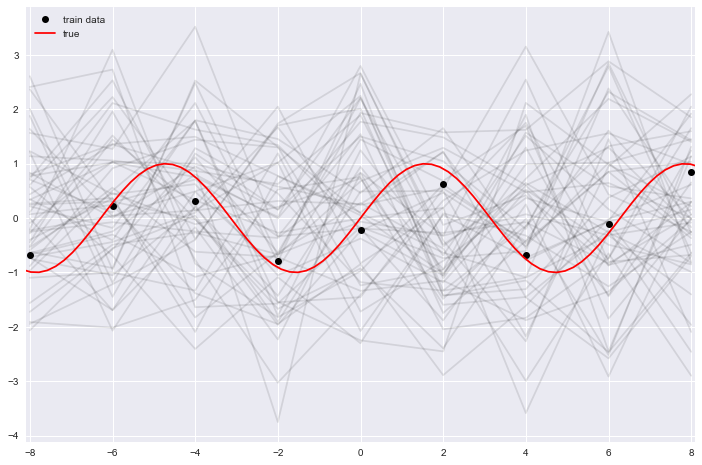It can often be wise to plot a couple of samples before starting a lengthy training process. These samples can be compared to the underlying data to see if the chosen model looks reasonable a priori. If the distribution of the target looks very different from the sampled distribution, it means it could not result from the assumed distribution. No matter how well you train, your model will never fit your data. In such a case, you probably need to find a better kernel function.

### Fitting¶

As always, to train the model, we call the fit method and pass the training data and targets as arguments:

In :
gpr.fit(X_train, y_train)

Re-initializing module.
Re-initializing criterion.
Re-initializing optimizer.
epoch    train_loss     dur
-------  ------------  ------
1        1.2771  0.0204
2        1.2650  0.0097
3        1.2533  0.0092
4        1.2416  0.0129
5        1.2312  0.0084
6        1.2211  0.0138
7        1.2111  0.0069
8        1.2017  0.0132
9        1.1932  0.0141
10        1.1850  0.0088
11        1.1771  0.0130
12        1.1698  0.0092
13        1.1632  0.0085
14        1.1570  0.0047
15        1.1511  0.0082
16        1.1456  0.0169
17        1.1408  0.0084
18        1.1363  0.0101
19        1.1320  0.0067
20        1.1281  0.0075

Out:
<class 'skorch.probabilistic.ExactGPRegressor'>[initialized](
module_=RbfModule(
(likelihood): GaussianLikelihood(
(noise_covar): HomoskedasticNoise(
(raw_noise_constraint): GreaterThan(1.000E-04)
)
)
(mean_module): ConstantMean()
(covar_module): RBFKernel(
(raw_lengthscale_constraint): Positive()
(distance_module): Distance()
)
),
)
Info: For GP regression, skorch does not perform a train/valid split by default. This is why you only see the train loss here, not the validation loss as usual. The reason for this decision is that a random split is most often not appropriate for GP regression. E.g. when you deal with a time series, random splitting would result in data leakage. Therefore, if you want validation scores, it is probably best to implement your own train_split or use skorch.helper.predefined_split if you already have split your data beforehand (see the example further below).

### Analyzing the trained model¶

Now that our model is trained, we can repeat the sampling process from above. As you can see, the samples fit the data much better now.

In :
samples = gpr.sample(X_train, 50)
samples = samples.detach().numpy()  # turn into numpy array

fig, ax = plt.subplots(figsize=(12, 8))
ax.plot(X_train, samples.T, color='k', alpha=0.1)
ax.plot(X_train, y_train, 'ko', label='train data')
ax.plot(X_valid, y_valid, 'r', label='true')
ax.set_xlim([-8.1, 8.1])
ax.legend();

/home/vinh/anaconda3/envs/skorch/lib/python3.7/site-packages/gpytorch/models/exact_gp.py:275: GPInputWarning: The input matches the stored training data. Did you forget to call model.train()?
GPInputWarning,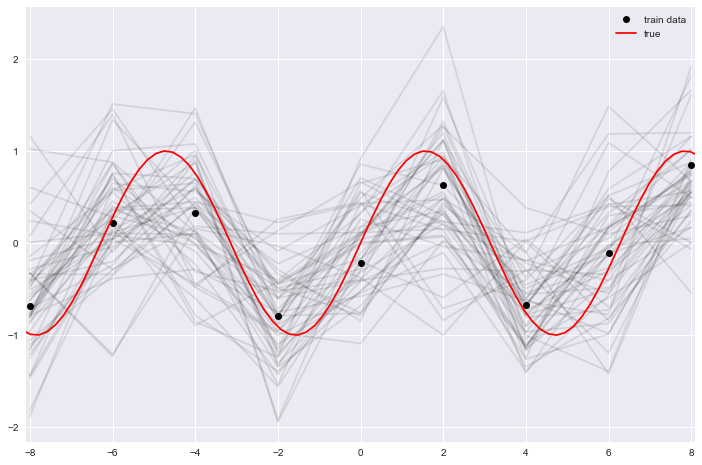Since the model represents a probability distribution, instead of just making point predictions as is most often the case for Deep Learning, we can use the distribution to give us confidence intervals. To do this, we can pass return_std=True to the predict call. This will return one standard deviation for the given data, which we can add/subtract from our prediction to get upper/lower confidence bounds:

In :
y_pred, y_std = gpr.predict(X_valid, return_std=True)

fig, ax = plt.subplots(figsize=(12, 8))
ax.plot(X_train, y_train, 'ko', label='train data')
ax.plot(X_valid, y_valid, color='red', label='true mean')
ax.plot(X_valid, y_pred, color='blue', label='predicted mean')
ax.fill_between(X_valid, y_pred - y_std, y_pred + y_std, alpha=0.5, label='+/- 1 std dev')
ax.legend()

Out:
<matplotlib.legend.Legend at 0x7f522f4309d0>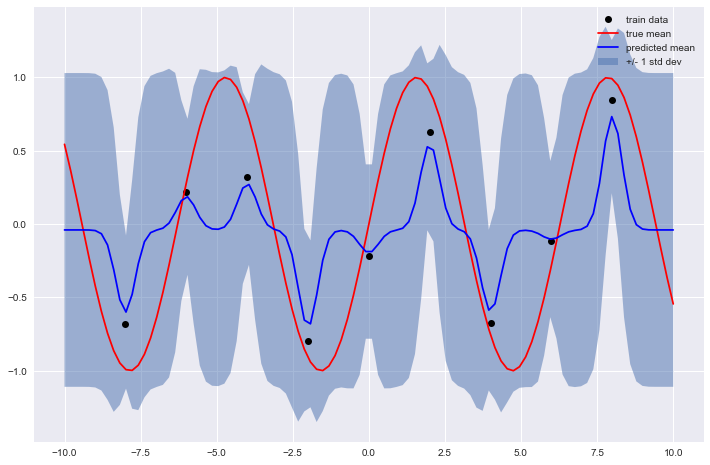As you can see, the confidence bounds are quite wide most of the time. Only at the training data points is the model more confident. This is exactly what we should expect: At the points where the model has seen some data, it is more confident, but between data points, it is less confident.

### More data¶

Below, we increase the sampling frequency from our sine function and train the same model again to show how a well fit model looks like.

In :
sampling_frequency = 2
X_train = torch.arange(-8, 9, 1 / sampling_frequency).float()
y_train = torch.sin(X_train) + torch.randn(len(X_train)) * 0.2

In :
gpr = ExactGPRegressor(
lr=0.1,
max_epochs=20,
batch_size=-1,
device=DEVICE,
)
gpr.fit(X_train.reshape(-1, 1), y_train)

  epoch    train_loss     dur
-------  ------------  ------
1        1.1546  0.0054
2        1.1222  0.0087
3        1.0879  0.0079
4        1.0535  0.0060
5        1.0188  0.0093
6        0.9833  0.0093
7        0.9474  0.0089
8        0.9114  0.0097
9        0.8754  0.0068
10        0.8395  0.0104
11        0.8038  0.0056
12        0.7684  0.0058
13        0.7337  0.0103
14        0.7000  0.0087
15        0.6673  0.0062
16        0.6357  0.0097
17        0.6054  0.0067
18        0.5762  0.0068
19        0.5479  0.0071
20        0.5200  0.0072

Out:
<class 'skorch.probabilistic.ExactGPRegressor'>[initialized](
module_=RbfModule(
(likelihood): GaussianLikelihood(
(noise_covar): HomoskedasticNoise(
(raw_noise_constraint): GreaterThan(1.000E-04)
)
)
(mean_module): ConstantMean()
(covar_module): RBFKernel(
(raw_lengthscale_constraint): Positive()
(distance_module): Distance()
)
),
)
In :
y_pred, y_std = gpr.predict(X_valid, return_std=True)

fig, ax = plt.subplots(figsize=(12, 8))
ax.plot(X_train, y_train, 'ko', label='train data')
ax.plot(X_valid, y_valid, color='red', label='true mean')
ax.plot(X_valid, y_pred, color='blue', label='predicted mean')
ax.fill_between(X_valid, y_pred - y_std, y_pred + y_std, alpha=0.5, label='+/- 1 std dev')
ax.legend()

Out:
<matplotlib.legend.Legend at 0x7f522b265fd0>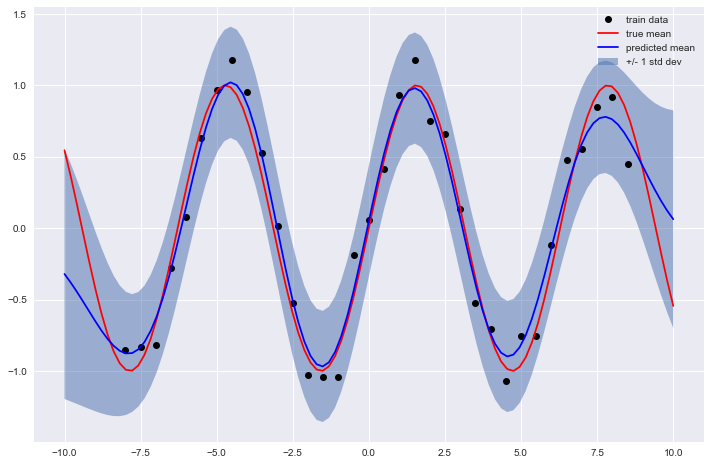Here we can see that the confidence intervals are much narrower than above, even between data points. This means that the model is quite confident in interpolating between data points. Notice, however, that the confidence bounds increase considerably at the left and right end, i.e. at values outside of the range of the training data. This means that the model is less confident in extrapolating which is typically a good thing.

### Confidence region¶

Another way skorch implements to quickly get the confidence interval is through the confidence_region method. This mirrors the method by the same name in GPyTorch. By default, it returns the lower and upper bound for 2 standard deviations, but this can be changed through the sigmas argument.

In :
lower, upper = gpr.confidence_region(X_valid, sigmas=2)

In :
plt.plot(upper, label='+2 std dev')
plt.plot(y_pred, label='mean')
plt.plot(lower, label='-2 std dev')
plt.legend();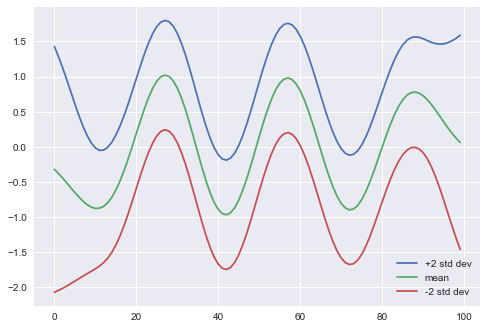As always, one of the advantages of using skorch is the sklearn integration. That means that we can plug our regressor into GridSearchCV et al. However, there is a caveat to this, as explained below. But first, let's set up the grid search.

In :
from sklearn.model_selection import GridSearchCV

In :
params = {
'lr': [0.01, 0.02],
'max_epochs': [10, 20],
}

In :
gpr = ExactGPRegressor(
RbfModule,
lr=0.1,
max_epochs=20,
batch_size=-1,
device=DEVICE,

train_split=False,
verbose=0,
)


We turn off skorch-internal train/validation split, since the grid search already performs the data splitting for us. We also set the verbosity level to 0 to avoid too many print outputs.

In :
search = GridSearchCV(gpr, params, cv=3, scoring='neg_mean_squared_error', verbose=1)


Since we deal with a regression task, we choose an appropriate scoring function, in this case mean squared error. Now let's start the grid search to see the problem mentioned above:

In :
search.fit(X_train, y_train)

Fitting 3 folds for each of 4 candidates, totalling 12 fits

[Parallel(n_jobs=1)]: Using backend SequentialBackend with 1 concurrent workers.
[Parallel(n_jobs=1)]: Done  12 out of  12 | elapsed:    0.9s finished

Out:
GridSearchCV(cv=3,
estimator=<class 'skorch.probabilistic.ExactGPRegressor'>[uninitialized](
module=<class '__main__.RbfModule'>,
),
param_grid={'lr': [0.01, 0.02], 'max_epochs': [10, 20]},
scoring='neg_mean_squared_error', verbose=1)

The grid search finished successfully and we found the best hyper-parameters:

In :
search.best_score_

Out:
-0.5268993576367696
In :
search.best_params_

Out:
{'lr': 0.01, 'max_epochs': 20}

## Regression with real world data¶

So far, we have only worked with toy data. To show how to work with real world data, we will reproduce an example from the GPyTorch docs:

https://docs.gpytorch.ai/en/stable/examples/01_Exact_GPs/Spectral_Delta_GP_Regression.html

This dataset contains the "BART ridership on the 5 most commonly traveled stations in San Francisco". "BART" is the "Bay Area Rapid Transit", i.e. public transit system in the San Francisco bay area.

### Getting the data¶

In :
path = os.path.join('datasets', 'BART_sample.pt')
if not os.path.isfile(path):
urllib.request.urlretrieve(url, path)

train_x, train_y, test_x, test_y = torch.load('../BART_sample.pt', map_location=DEVICE)


We need to scale the input data a bit, following the tutorial:

In :
train_x_min = train_x.min()
train_x_max = train_x.max()

X_train = train_x - train_x_min
X_valid = test_x - train_x_min


The target data also needs to be scaled. However, contrary to the tutorial, we will use sklearn's StandardScaler for this. Although it performs the same transformation as in the tutorial, it allows us to easily inverse transform the targets back to their original scale, which will be useful later.

In :
from sklearn.preprocessing import StandardScaler

In :
# We need to transpose here because the target data is sequence x sample
# but we want to scale over the samples, not over the sequence.
y_scaler = StandardScaler().fit(train_y.T)
y_train = torch.from_numpy(y_scaler.transform(train_y.T).T).float()
y_valid = torch.from_numpy(y_scaler.transform(test_y.T).T).float()

In :
print(X_train.shape, y_train.shape, X_valid.shape, y_valid.shape)

torch.Size([5, 1440, 1]) torch.Size([5, 1440]) torch.Size([5, 240, 1]) torch.Size([5, 240])


### Defining the module¶

The module is effectively the same as in the tutorial.

In :
class SpectralDeltaGP(gpytorch.models.ExactGP):
def __init__(self, X, y, likelihood, num_deltas, noise_init=None):
super(SpectralDeltaGP, self).__init__(X, y, likelihood)
self.mean_module = gpytorch.means.ConstantMean()
base_covar_module = gpytorch.kernels.SpectralDeltaKernel(
num_dims=X.size(-1),
num_deltas=num_deltas,
)
self.covar_module = gpytorch.kernels.ScaleKernel(base_covar_module)

def forward(self, x):
mean_x = self.mean_module(x)
covar_x = self.covar_module(x)
return gpytorch.distributions.MultivariateNormal(mean_x, covar_x)


### Defining the likelihood¶

Here we show an example of using a non default likelihood. We wrap the initialization of the likelihood inside a function because we want to use some specific methods, such as register_prior. This function can be passed as the likelihood parameter to ExactGPRegressor.

In :
def get_likelihood(
noise_constraint=gpytorch.constraints.GreaterThan(1e-11),
noise_prior=gpytorch.priors.HorseshoePrior(0.1),
noise=1e-2,
):
likelihood = gpytorch.likelihoods.GaussianLikelihood(
noise_constraint=noise_constraint,
)
likelihood.register_prior("noise_prior", noise_prior, "noise")
likelihood.noise = noise
return likelihood

In :
gpr = ExactGPRegressor(
SpectralDeltaGP,
module__num_deltas=1500,
module__X=X_train if DEVICE == 'cpu' else X_train.cuda(),
module__y=y_train if DEVICE == 'cpu' else y_train.cuda(),

likelihood=get_likelihood,

lr=2e-4,
max_epochs=50,
batch_size=-1,
device=DEVICE,
)


Again, as explained above, we additionally need to pass X and y to the module and we need to set the batch size to -1, since we still deal with exact GPs.

### Training the model¶

In :
# This context manager ensures that we dont try to use Cholesky. This is
# in following with the tutorial.
with gpytorch.settings.max_cholesky_size(0):
gpr.fit(X_train, y_train)

  epoch    train_loss     dur
-------  ------------  ------
1        2.7906  2.0871
2        2.1317  1.9986
3        1.6960  3.0301
4        1.4273  3.0680
5        1.2657  3.5300
6        1.1890  2.5725
7        1.1178  2.0474
8        1.0708  2.0823
9        1.0580  2.0268
10        1.0362  2.0235
11        1.0192  1.8889
12        0.9654  1.8637
13        0.9529  1.8471
14        0.9309  1.9733
15        0.9019  2.1043
16        0.9180  1.9568
17        0.8743  1.9917
18        0.8694  1.9267
19        0.8587  2.1423
20        0.8542  1.8739
21        0.8462  1.8677
22        0.8018  2.0562
23        0.7965  2.0123
24        0.7790  2.0773
25        0.7978  2.1713
26        0.7567  2.2295
27        0.7393  2.2569
28        0.7321  2.1236
29        0.7017  1.8720
30        0.6973  1.6813
31        0.7043  1.9117
32        0.7009  2.1291
33        0.6733  2.0217
34        0.6713  2.1509
35        0.6629  1.8898
36        0.6720  1.7500
37        0.6548  1.8919
38        0.6356  1.8814
39        0.6187  2.0170
40        0.6403  2.2680
41        0.6381  1.9118
42        0.6263  2.0442
43        0.6327  2.0453
44        0.6357  1.8428
45        0.5957  1.7891
46        0.5961  1.8859
47        0.6135  1.8681
48        0.6238  1.7084
49        0.5978  1.7232
50        0.5919  1.7004


### Analyzing the trained model¶

Again, let's plot the predictions and the standard deviations for our 5 time series and see how well the model learned.

In :
%%time
y_pred, y_std = gpr.predict(X_valid, return_std=True)

CPU times: user 26.5 s, sys: 6.6 s, total: 33.1 s
Wall time: 8.29 s


Here we make use of our StandardScaler to scale the targets back to their original scale. Remember to also scale the standard deviations.

In :
y_pred = y_scaler.inverse_transform(y_pred.T).T
y_std = y_scaler.inverse_transform(y_std.T).T

In :
def plot_bart(ax, X_train, y_train, X_valid, y_valid, y_pred, y_std):
lower = y_pred - y_std
upper = y_pred + y_std

ax.plot(X_train, y_train, 'k*', label='train')
ax.plot(X_valid, y_valid, 'r*', label='valid')
# Plot predictive means as blue line
ax.plot(X_valid, y_pred, 'b')
# Shade between the lower and upper confidence bounds
ax.fill_between(X_valid, lower, upper, alpha=0.5, label='+/- 1 std dev')
ax.tick_params(axis='both', which='major', labelsize=16)
ax.tick_params(axis='both', which='minor', labelsize=16)
ax.set_ylabel('Passenger Volume', fontsize=16)
ax.set_xticks([])
return ax

In :
fig, axes = plt.subplots(5, figsize=(14, 28))
y_train_unscaled = y_scaler.inverse_transform(y_train.T).T
y_valid_unscaled = y_scaler.inverse_transform(y_valid.T).T
for i, ax in enumerate(axes):
ax = plot_bart(
ax,
X_train[i, -100:, 0],
y_train_unscaled[i, -100:],
X_valid[i, :, 0],
y_valid_unscaled[i],
y_pred[i],
y_std[i],
)
ax.set_title(f"Station {i + 1}", fontsize=18)

ax.set_xlabel('hours', fontsize=16)
plt.xlim([1250, 1680])
plt.tight_layout()